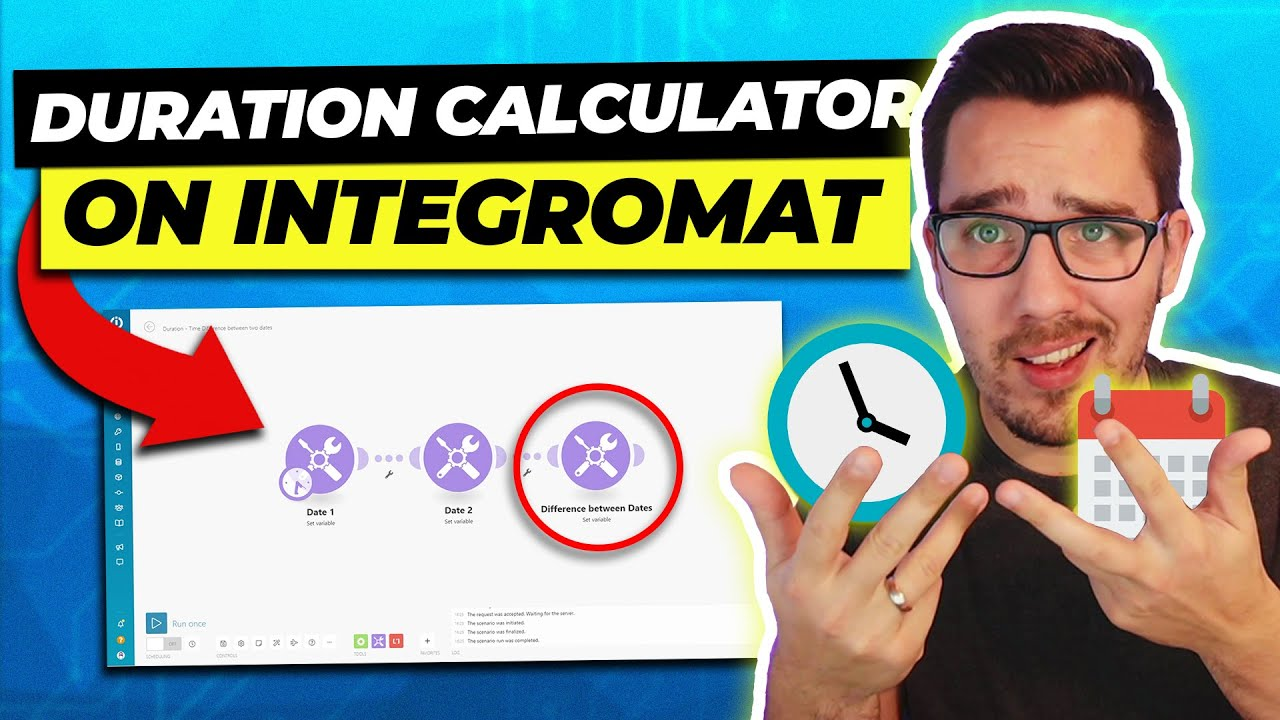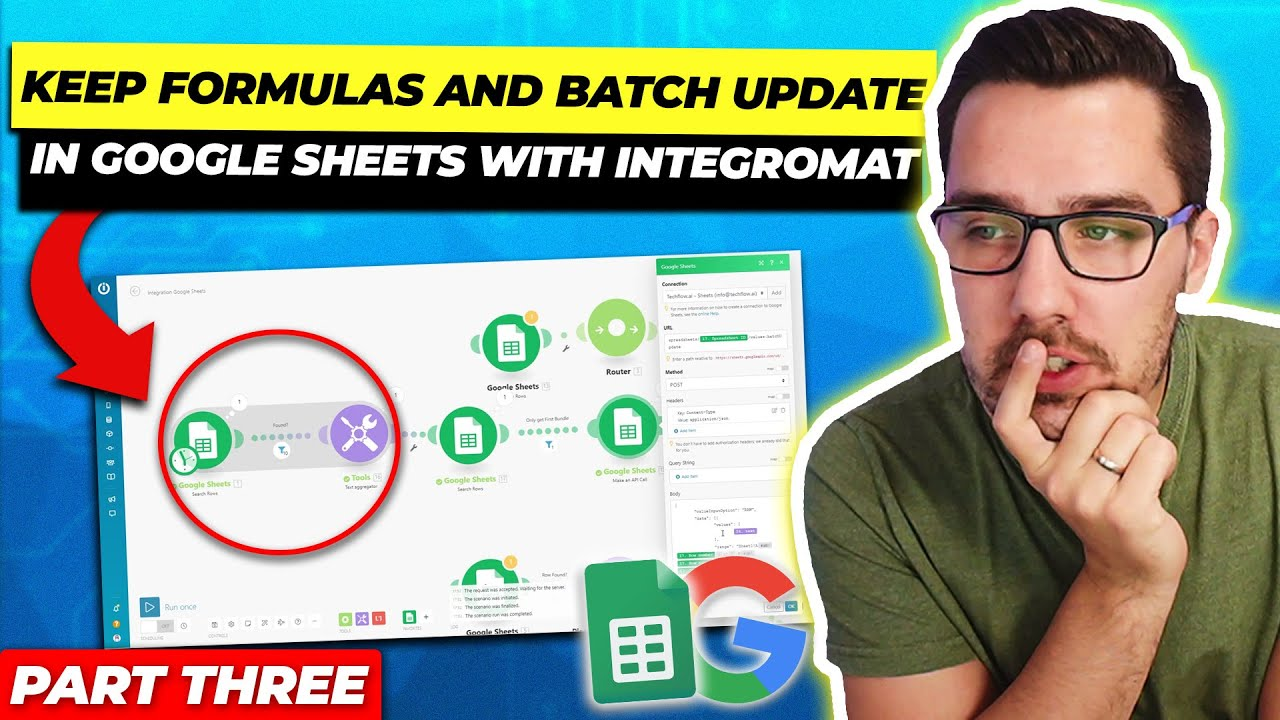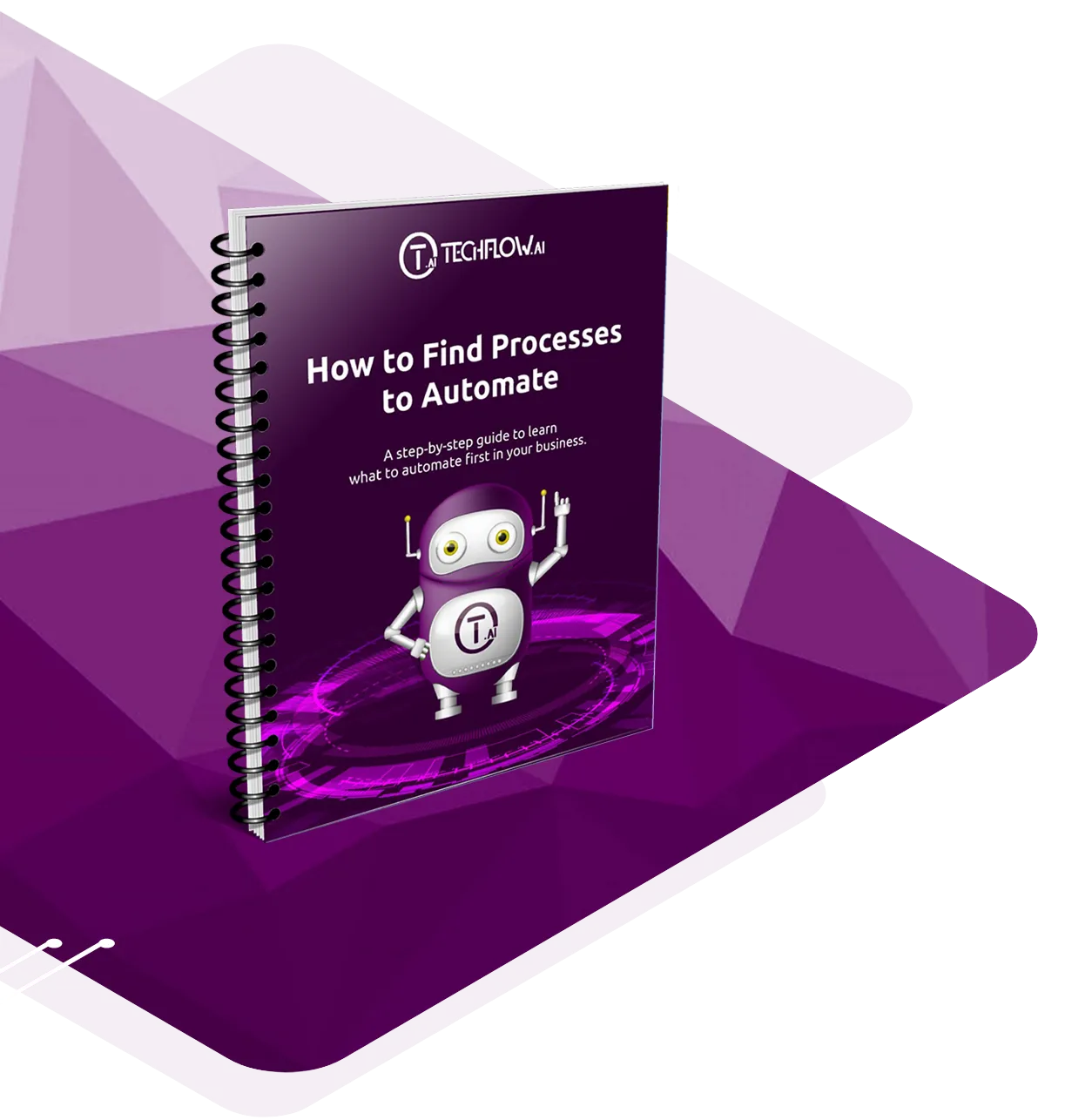# Calculate Duration between two Dates in Integromat 2021

When working with Integromat, you most likely also work with dates, or you have to work with dates and dates can be really confusing. In this blog, you will learn how you can calculate the difference between two dates to know the days in between or even the days, the hours, the minutes and the seconds.When working with Integromat, you most likely also work with dates, or you have to work with dates and dates can be really confusing. In this blog, you will learn how you can calculate the difference between two dates to know the days in between or even the days, the hours, the minutes and the seconds.

We look at it using an example, and I will explain to you all the functions that you will need and how to set it up.

I've prepared this sample scenario.

We have Date 1, which is the 8th of September, and we have Date 2, which is the 9th of April.

We want to see the difference between these two dates. You could theoretically do ‘Date 1  minus Date 2’. But when you run this once, you see the time difference, it's just empty.

Why is that? Because it understands this as a text string. And for a text string, you can't make a calculation. You can't say day one minus day two. We have to parse the date first. So we use the parseDate function.

The parseDate function basically transforms the string into an actual date, and then you can do more things with it.

Add the parseDate function in the variable value on both dates.

If we run it once again, we see it still looks exactly the same. But the output of this function is now actually, time, that is, in milliseconds. That's basically the time difference between these two dates in milliseconds.

But how do we show the time difference between these two dates in seconds?

Let’s format them into seconds. We can do this using the formatDate function.

As the value for the format, we use the uppercase X, which means seconds. You could also use the lowercase x, which is milliseconds, and that will give the same output as if you calculated the difference right away.

Run it once and below are the results in milliseconds and seconds.

With seconds, you can easily calculate everything. You could also do it in milliseconds. You just have to divide it by 1000, and then you get the actual seconds.

The next would be to calculate the days. How many days are there between two dates?

There's this handy function called the round function.

We have date one minus date two. Then we divide it by 1000. Divide it by 60, divide it by 60 again, and lastly, divide it by 24. Why is that? Because we have 24 hours a day, 60 minutes an hour, 60 seconds a minute, and 1000 milliseconds a second.

Since the output is in milliseconds, we have to divide by 1000 to get the value in seconds.  Then we divide it by 60 to get the value in minutes. Then we divide it by 60 again to get the value in  hours, and then we divide it by 24 to get the actual number of days.

So the output is 152, as you can see here.

Check the results over on Google and see.

How to calculate for hours, minutes, and seconds.

Now we want to get hours, minutes and seconds split up.

Divide the date difference first by 3600 to get the hours, and we use the floor function to round it down to the full hour, because we don't want, like, 3600.57 hours because that doesn't make sense. So we divide by 3600.

For the minutes, we have the floor function as well. We use the value that we had before this same variable.  Then we use modulus 3600, which means it is divided by 3600 and then give back the rest. Divide that value by 60.  That gives us the minutes.

Do the same for seconds. Use modulus 3600, which gives the rest of the first function of the hours. Then again, modulus 60, which gives the rest of the minutes. And that is the second.

So now running that again, it is 3656 hours, 47 minutes and 45 seconds.

Now, let’s make this a bit more complicated, shall we? Let’s add in the days.

The main difference is we divide it by 86,400, because 86,400 is how many seconds one day has.

Divide the time difference in seconds by 86,400, then use the floor function to round it down to the full number to get the number of days.

Use the modulus function again to get the rest of it and divide it by 3600 like we did before to get the hours.

Use the modulus two times again, with 86,400 to get the days, then 3600 to get the hours and then divided by 60 to have it in minutes.

Last but not the least, do the same with modulus 86,400, modulus 3,600 and modulus 60 to get the seconds.

The output is 152 days, 8 hours, 47 minutes and 45 seconds.  That's exactly what the time difference is between these two dates.

You can use this however you want to, and however you need it. If you want to say okay, time difference needs to be exactly 30 days and 50 seconds, then you can set up a filter that the days and the seconds should be above that value or the total seconds should be above that value.  You can also save the result into a custom field and use it further.

I hope the explanation was helpful for you because I know times are quite hard to grasp. I will provide more blogs about times, working with times and timestamps and working with different time zones in the future.

If you think that these Integromat functions that I've used here look cool but you have no idea how they work, then I suggest you look into the Integromat functions cheat sheet where I have over 45 pages of Integromat functions explained. You can download it. It's in PDF and it's fully searchable. You can just type whatever keyword you want, and it will search through the whole PDF and guide you directly to the correct place.  You will have copy and paste ready functions in there with an explanation and some examples that you can use inside your scenarios.

## Key Takeaway

Integromat functions are super helpful.  They provide a lot of value, as I have just recently demonstrated, and they enhance your automation without using any kind of operation. Operation costs money, and you save massive operations using Integromat functions. The thing about using Integromat is that you can have a high degree of control over your processes and have them perform without any intervention from you. Consider the many areas where integrating your business processes can lead to significant benefits. You can use Integromat when handling & automating tasks for operations management, human resources and delivery services, to name a few.

Learn more about what else you can automate in Integromat by taking the Integromasters course. It's an interactive course where you will be able to learn the basics of Integromat and get to know it's most used features. There will be several lessons for you to learn, along with some quizzes to help you understand the information. It's an affordable way to learn what you have always wanted to know about Integromat!#### Google Sheets Automation Tips HACK | Keep Formulas and Batch Updates (Part 03)

In Part 03 you'll learn a HACK how to keep formulas alive when adding new rows to Google Sheets using Integromat as well as how to batch update multiple rows...

Guides, Hacks & Tutorials#### How to use the Make (formerly Integromat) Webhook

Integromat webhooks are a great way to connect any service to start your automations on Make instantly when something happens on the other platform.

Guides, Hacks & Tutorials#### How to Boost Productivity with Workflow Automation

More and more organizations are automating repetitive tasks to make their workflows more efficient, improving employee productivity.

Guides, Hacks & Tutorials## Stop Wasting Time

Feeling frustrated from spending too much time on tasks you don’t enjoy?

You don’t have to be the one doing it.